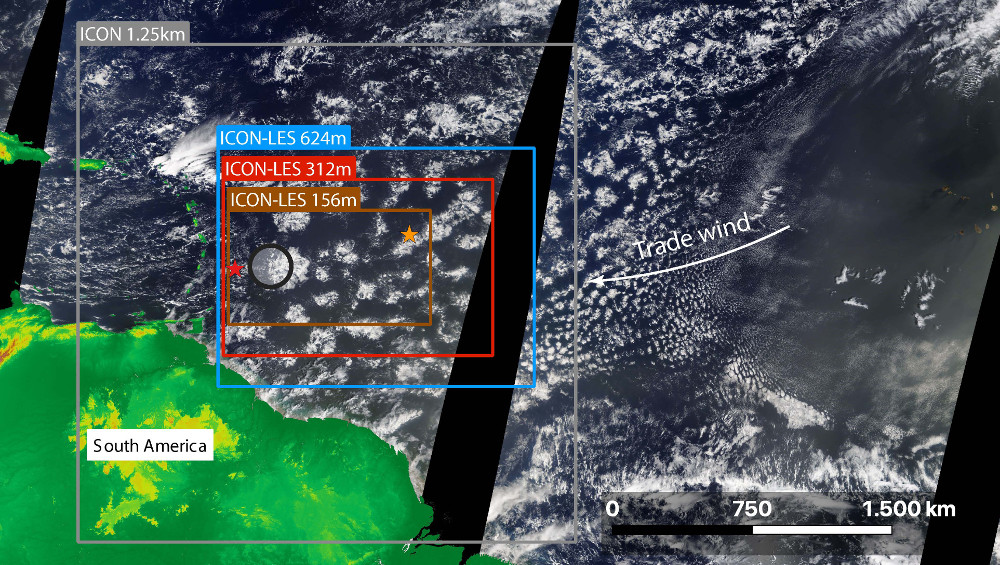# ICON-LES#

## Setup#

These ICON Large-eddy simulations (LES) presented here consist of three different domains that have different spatial and temporal extends depending on their horizontal grid-spacing. Fig. 2 shows the extend of the different simulations.Fig. 2 Overview of simulation domains. The location of the Barbados Cloud Observatory (BCO) and the NTAS buoy are marked with a red star at the western and eastern part of the domain, respectively. For a sense of scale, the MODIS image of February 12 is shown with landmasses colored in green to brown depending on height. Satellite image source: NASA Worldview.#

Fig. 2 illustrates further the extend of the ICON Storm-resolving simulation (SRM) that provides the boundary conditions for the ICON-624m run. ICON-312m and ICON-156m are both one-way nested in the respective coarser resolution run.

## Availability of simulation output#

Note

The currently available ICON-LES output is shown in the following:## Output description#

There are two main experiments: LES_CampaignDomain_control and LES_CampaignDomain_highCCN. These experiments only distinguish themselves by the prescribed cloud condensation nuclei (CCN) concentration, respectively 1700 cm$$^{-3}$$ and 130 cm$$^{-3}$$. DOM01 refers to the 624m run, while DOM02 refers to the 312m nest. As the variable names suggest, the surface entries contain the surface variables. rttov refers to forward simulated synthetic satellite images and meteogram output is available at different locations using the entry format meteogram_<location>_<domain>. The 2D and 3D radiation fields and fluxes are referenced with radiation.

## Visualization#

The simulation output is mostly available on its natural, icosahedral grid but some output, like the rttov brightness temperatures, have been regridded onto a regular lat-lon grid. The following section shows how these different outputs can be visualized and explored.

### Regular grid#

import eurec4a
import xarray as xr
import numpy as np
import pandas as pd

import matplotlib.pylab as plt

import cartopy.crs as ccrs
import cartopy.feature as cf


#### Selecting output#

The simulation output is indexed in the EUREC4A Intake catalog and can be easily queried:

cat = eurec4a.get_intake_catalog()

print(data)
variable = "synsat_rttov_forward_model_1__abi_ir__goes_16__channel_7"  #choose one of the once below
da = data[variable].sel(time='2020-02-08 12:00:00')

<xarray.Dataset>
Dimensions:                                                   (lat: 760,
lon: 1220,
time: 5903)
Coordinates:
* lat                                                       (lat) float64 7...
* lon                                                       (lon) float64 -...
* time                                                      (time) datetime64[ns] ...
Data variables:
synsat_rttov_forward_model_1__abi_ir__goes_16__channel_1  (time, lat, lon) float32 dask.array<chunksize=(12, 760, 1220), meta=np.ndarray>
synsat_rttov_forward_model_1__abi_ir__goes_16__channel_2  (time, lat, lon) float32 dask.array<chunksize=(12, 760, 1220), meta=np.ndarray>
synsat_rttov_forward_model_1__abi_ir__goes_16__channel_3  (time, lat, lon) float32 dask.array<chunksize=(12, 760, 1220), meta=np.ndarray>
synsat_rttov_forward_model_1__abi_ir__goes_16__channel_4  (time, lat, lon) float32 dask.array<chunksize=(12, 760, 1220), meta=np.ndarray>
synsat_rttov_forward_model_1__abi_ir__goes_16__channel_5  (time, lat, lon) float32 dask.array<chunksize=(12, 760, 1220), meta=np.ndarray>
synsat_rttov_forward_model_1__abi_ir__goes_16__channel_6  (time, lat, lon) float32 dask.array<chunksize=(12, 760, 1220), meta=np.ndarray>
synsat_rttov_forward_model_1__abi_ir__goes_16__channel_7  (time, lat, lon) float32 dask.array<chunksize=(12, 760, 1220), meta=np.ndarray>
Attributes:
CDI:                       Climate Data Interface version 1.9.10 (https:/...
CDO:                       Climate Data Operators version 1.9.10 (https:/...
Conventions:               CF-1.6
comment:                   Hauke Schulz (m300408) on l30466 (Linux 4.18.0...
history:                   Fri Apr 22 18:15:09 2022: cdo -r -P 30 -f nc4 ...
institution:               Max Planck Institute for Meteorology
references:                see MPIM/DWD publications
source:                    icon-nwp\tgit@gitlab.dkrz.de:icon/icon-nwp.git...
title:                     ICON simulation


#### Setting the scene#

central_longitude = -53.54884554550185
central_latitude = 12.28815437976341
satellite_height = 8225469.943160511

vmin = 270
vmax = 300
cmap = "RdBu_r"


#### Projection and plotting#

The regular grid data can also be plotted with a simple da.plot(), but more steps are involved for projections and some tweeks for faster plotting that come especially handy for the plotting of data on the icosahedral grid shown in the next section.

projection = ccrs.NearsidePerspective(central_longitude=central_longitude, central_latitude=central_latitude, satellite_height=satellite_height)

lats, lons = np.meshgrid(data.lat,data.lon)
coords = projection.transform_points(
ccrs.Geodetic(),
lons.T.flatten(),
lats.T.flatten(),
)

fig, ax = plt.subplots(subplot_kw={"projection": projection})
fig.canvas.draw_idle()

artist = dsshow(
pd.DataFrame({
"val": da.values.flatten(),
"x": coords[:, 0],
"y": coords[:, 1],
}),
vmin=vmin,
vmax=vmax,
cmap=cmap,
ax=ax,
)

fig.colorbar(artist, label=f"{da.long_name} / {da.units}");

/usr/share/miniconda/envs/how_to_eurec4a/lib/python3.8/site-packages/IPython/core/pylabtools.py:151: UserWarning: constrained_layout not applied because axes sizes collapsed to zero.  Try making figure larger or axes decorations smaller.
fig.canvas.print_figure(bytes_io, **kw)### Icosahedral grid#

For the visualization of the icosahedral grid, and interactive version exists that relies on gridlook and is shown below for the surface values of LES_CampaignDomain_control and DOM01. Try it out!

Static views can be exported within the app and plotted separatedly. Here is an example for the 2m temperature that build on top of the plotting routine of the regular grid.

# Lazy loading of output and grid

central_longitude = -53.54884554550185
central_latitude = 12.28815437976341
satellite_height = 8225469.943160511

vmin = 294.6328125
vmax = 299.84375
cmap = "RdBu_r"
variable = "t_2m"

da = data[variable].sel(time='2020-02-08 12:00:00')

projection = ccrs.NearsidePerspective(central_longitude=central_longitude, central_latitude=central_latitude, satellite_height=satellite_height)

coords = projection.transform_points(
ccrs.Geodetic(),
)

fig, ax = plt.subplots(subplot_kw={"projection": projection})
fig.canvas.draw_idle()

artist = dsshow(
pd.DataFrame({
"val": da.values,
"x": coords[:, 0],
"y": coords[:, 1],
}),
vmin=vmin,
vmax=vmax,
cmap=cmap,
ax=ax,
)

fig.colorbar(artist, label=f"{da.long_name} / {da.units}");

/usr/share/miniconda/envs/how_to_eurec4a/lib/python3.8/site-packages/IPython/core/pylabtools.py:151: UserWarning: constrained_layout not applied because axes sizes collapsed to zero.  Try making figure larger or axes decorations smaller.
fig.canvas.print_figure(bytes_io, **kw)## Questions and further information#

Questions and issues with this dataset should be raised at the GITLAB-Repository to encourage an open discussion and share experiences. The repository is also the place where further information about these simulations can be found.